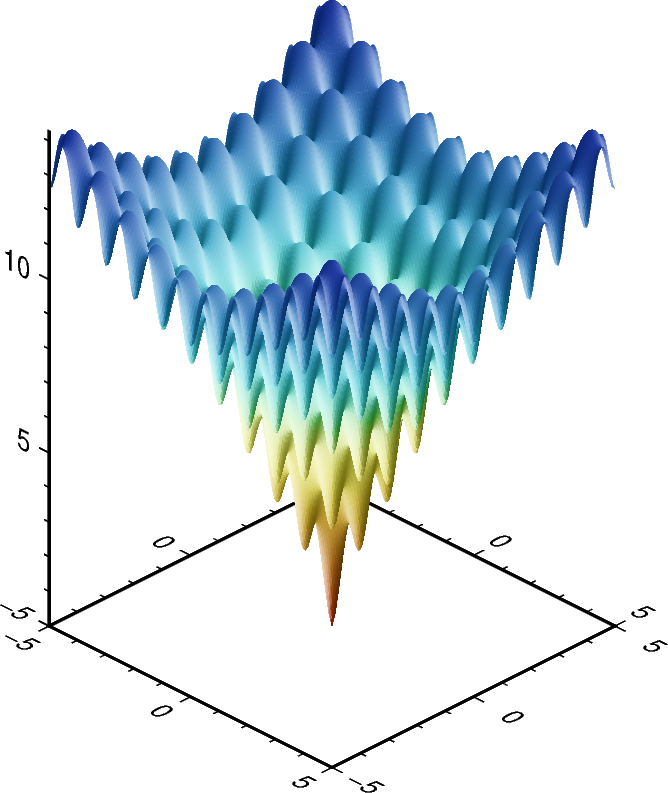# Plotting a surface¶

The pygmt.Figure.grdview method can plot 3-D surfaces with surftype="s". Here, we supply the data as an xarray.DataArray with the coordinate vectors x and y defined. Note that the perspective argument here controls the azimuth and elevation angle of the view. We provide a list of two arguments to frame — the second argument, prepended with "z", specifies the $$z$$-axis frame attributes. Specifying the same scale for the projection and zcale arguments ensures equal axis scaling. The shading argument specifies illumination; here we choose an azimuth of 45° with shading="+a45".Out:

<IPython.core.display.Image object>


import pygmt
import numpy as np
import xarray as xr

# Define an interesting function of two variables, see:
# https://en.wikipedia.org/wiki/Ackley_function
def ackley(x, y):
return (
-20 * np.exp(-0.2 * np.sqrt(0.5 * (x ** 2 + y ** 2)))
- np.exp(0.5 * (np.cos(2 * np.pi * x) + np.cos(2 * np.pi * y)))
+ np.exp(1)
+ 20
)

# Create gridded data
INC = 0.05
x = np.arange(-5, 5 + INC, INC)
y = np.arange(-5, 5 + INC, INC)
data = xr.DataArray(ackley(*np.meshgrid(x, y)), coords=(x, y))

fig = pygmt.Figure()

# Plot grid as a 3-D surface
SCALE = 0.2  # [inches]
fig.grdview(
data,
frame=["a5f1", "za5f1"],
projection=f"x{SCALE}i",
zscale=f"{SCALE}i",
surftype="s",
cmap="roma",
perspective=[135, 30],  # Azimuth southeast (135°), at elevation 30°